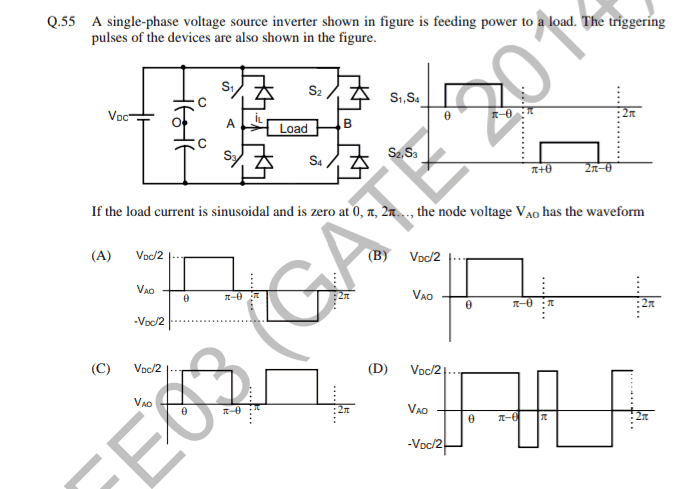# Single Phase Inverter Waveform

• Engineering
Homework Helper
Gold Member
Homework Statement:
Find the pole voltage waveform Vao.
Relevant Equations:
Waveform plotting based on KCL and KVL.The answer given is d).

Seeing the problem statement, it can be concluded that the load has some inductance, and when the switches S1, S2, S3 and S4 are off, conduction takes place through the anti-parallel body diodes (freewheeling action).

Seeing the triggering pulse waveforms, S1-S4 ON, Vao= +Vdc/2
S1-S4 OFF, D2-D3 ON, Vao = -Vdc/2.
This is only seen in d). So I can eliminate a), b) and c).

But I find d) incorrect as well.
The load current at "wt=pi" is 0 as per the problem statement. This means neither the switches nor the diodes are conducting at wt=pi. So how can Vao jump from -Vdc/2 to +Vdc/2 at wt= pi?
Shouldn't it be floating at some unknown value?

What am I missing here?
Any help is appreciated.

## Answers and Replies

DaveE
Science Advisor
Gold Member
Since the load current is sinusoidal it is changing polarity when it crosses zero at pi. This will cause the commutating diode pairs to switch, that is why the voltage changes. I suppose at the precise instant the current changes you could argue it's floating, but that's virtually zero duration.

•cnh1995
Homework Helper
Gold Member
Since the load current is sinusoidal it is changing polarity when it crosses zero at pi.
Yes, I agree with your reasoning.
Looks like the load is RLC type, and the voltage across C at wt=pi is responsible for diode pair switching.
I believe I was overthinking; it wasn't necessary to know the type of load and its components . Only 'the current is sinuoidal' part is enough to answer this question.

Thanks a lot! I have to stop overthinking.DaveE
Science Advisor
Gold Member
In SMPS designs where the inductor current is discontinuous (i.e. the energy stored is all used in each cycle), then you do see the switch voltages floating at times. In practice this almost always looks like part of a sinusoidal resonance of parasitic L & C. So your intuition wasn't too far off.

edit: Oops, inductor current is never discontinuous. I should have said load, or input current.

•cnh1995